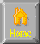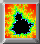```
TOPOLOGY

Topology = Idealization of space
euclidean
noneuclidean
analytic
affine
projective geometry

Klein-Erlanger Program -> Transformation key! not object!
1. Determine topological equivalence of spaces (Homeomorphics)
2. Determine if space x has fixed point
||
\/
Topology
Classify Topology spaces - equivalence classes/cannonical instances
1. Spheres w/ g (genus) handles (All closed surface topologically equivalent to)
2. Cross caps (All non-orientable closed surface topologically equivalent to)
\/
Mobius band - open surface w/ 1 edge ; non-orientable
Cross cap = sew mobius band into sphere w/ hole in it

||
\/

Fixed Points
Brouwer fix point theorem
X= topological space compact + convex
Compact = finite dim topo space bounded w/ all points of boundary
Convex = pt-to-pt enclosure (triangle,circle but not horshoe,torus)
f = continuous map of X to itself then f has fixed pt in X,
i.e. there exists x* in X s.t. f(x*)=x*
Sperner's Lemma
Chop up space
|    ._.         A/____|
|   /A  \   _____--    |
|  /   __\_-    /      |  lim del X find fixed point
|------   \____/ B     |
|                      |
+----------------------+
A  A  A   B  B   A  A

1. Cyclones - brouwer's fix pt -> can't comb wind to eliminate pt of zero vertical movement
2. Hohmann path - find map f whose fixed pts  = solution optimal to newton eqn (hohmann path)
3. Equilibria class divisions - occupational mobility
4. Perron-Frobenius Theorem Ar=r Team score vector, pt of constancy
5. Adams (humanitraianistic economics)- L. Walrus (supply/Demand) - V. Neumann(equilib HI out Lo\$, HI rate)
- Arrow (Gen equilib thy)
6.       /\
/_p\  po->p1 in economic brouwer this is equilibrium pt
/p   \  p1 s.t. apply T to p0 get to p1.
/______\

```HomeNext ... Critical Points